# 1.6. Calculating coupler coefficients¶

A MZI Lattice filter uses a set of identical delay lines, or delay lines that are an integer multiple of a unit delay $$L$$ which resembles just like in discrete Finite Impulse Response (FIR) filter.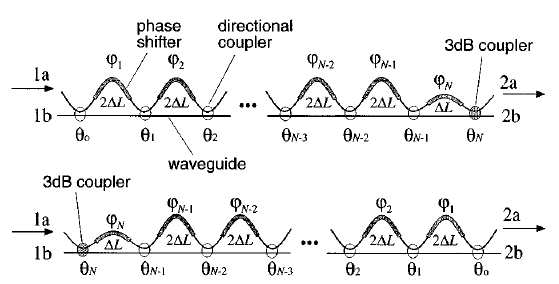Mathematical tools have been developed to approximate an ideal filter function on a discrete set of zeros that can be synthesized through an FIR filter.

The zeros coming out of such a design method that can be translated into a set of coupling coefficients through a method put forward by Jinguji and Kawashi in the paper Synthesis of Coherent Two-Port Lattice-Form Optical Delay-Line Circuit

A special class of FIR filters are Optical Half-Band Filters which have the property of having a symmetric passband and crossband. This property makes them very practical to be used in cascaded demultiplexers. The half-band lattice filters uses delays of both $$L$$ and $$2L$$. This approach can raise the order of the filter with fewer directional couplers.

In Optical Half-Band Filters the coupling coefficients of optical half band filters are computed for a Chebychev window and a window yielding a maximally flat passband as a function of center wavelength and trace template.

In the code below, we use the results from the paper to calculate the coupling coefficients and delay lengths.

from si_fab import all as pdk
import numpy as np
from lattice_utils import get_mzi_delta_length_from_fsr, get_length_pi, flip_couplings_and_delays

tt = pdk.SiWireWaveguideTemplate()
# Series of power couplings and delay lengths for optical halfband filters
center_wavelength = 1.5
length = get_mzi_delta_length_from_fsr(trace_template=tt, center_wavelength=center_wavelength, fsr=0.01)
length_pi = get_length_pi(trace_template=tt, center_wavelength=center_wavelength)

# Maximally Flat

# N = 2
power_couplings_2 = np.sin(np.array([0.0833, 0.333, 0.25]) * np.pi)**2
delay_lengths_2 = [2*length-length_pi, length]
print(power_couplings_2)
# N = 3
power_couplings_3 = np.sin(np.array([0.4664, 0.1591, 0.3755, 0.25]) * np.pi)**2
delay_lengths_3 = [2*length, 2*length-length_pi, length]
print(power_couplings_3)
# N = 4
power_couplings_4 = np.sin(np.array([0.3720, 0.2860, 0.4547, 0.1187, 0.25]) * np.pi)**2
delay_lengths_4 = [2*length-length_pi, 2*length, 2*length-length_pi, length]
print(power_couplings_4)

# Chebychev
# N = 4
power_couplings_4c = np.sin(np.array([0.3498, 0.2448, 0.4186, 0.0797, 0.25]) * np.pi)**2
delay_lengths_4c = [2*length-length_pi, 2*length, 2*length-length_pi, length]
print(power_couplings_4c)

[ 0.06693495  0.74909255  0.5       ]
[ 0.98889893  0.22970362  0.85466236  0.5       ]
[ 0.84682665  0.61213538  0.97988305  0.13273214  0.5       ]
[ 0.79338407  0.48366662  0.93601755  0.0613934   0.5       ]


## 1.6.1. Transforming the filter¶

When the required directional coupler power $$p$$ couplings that come out of the synthesis are close to 1 the directional couplers that implement such a coupling tend to be longer and more dispersive. This is shown in the figures below demonstrating a directional couplers with length 0.02 and 0.98.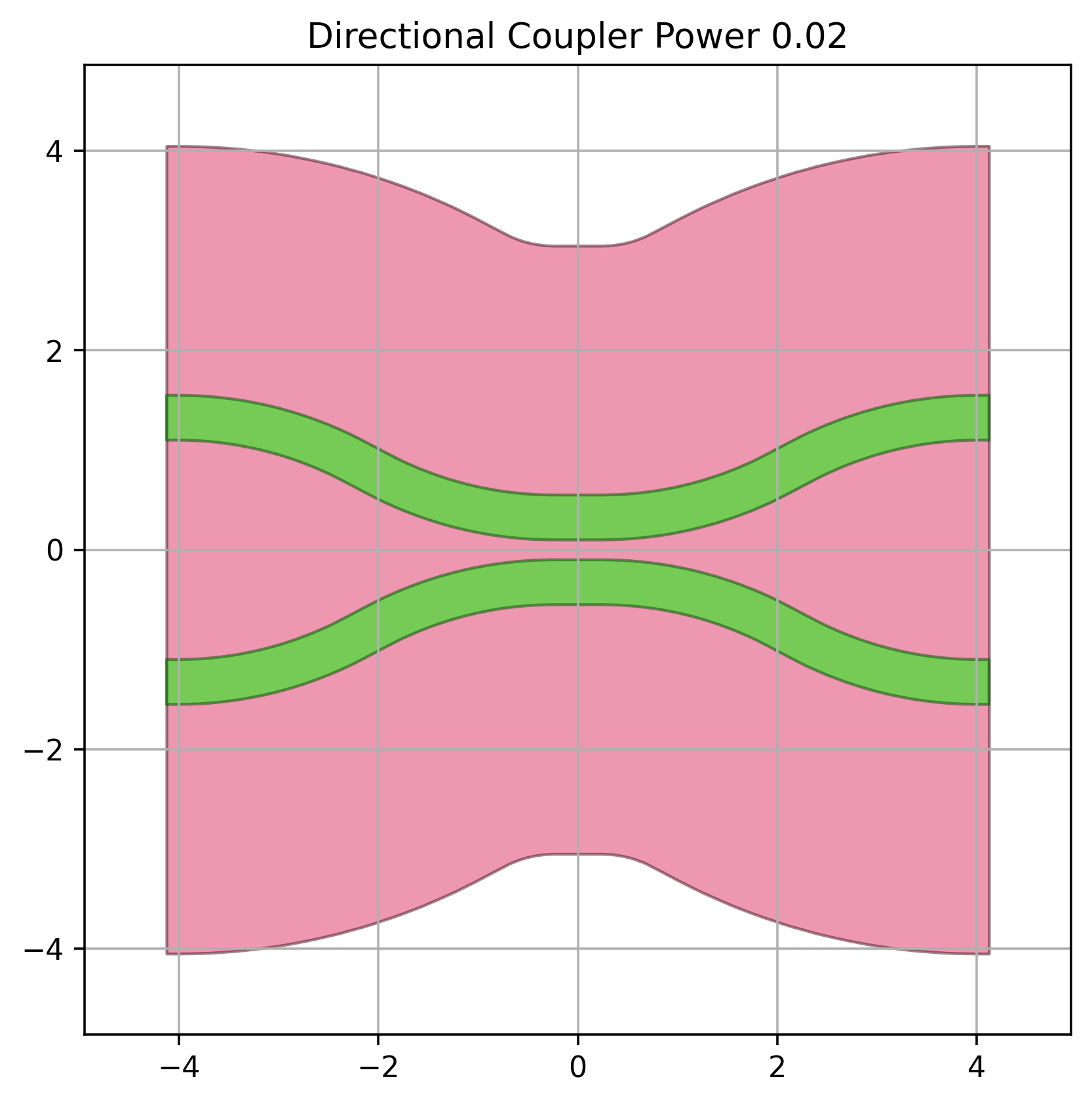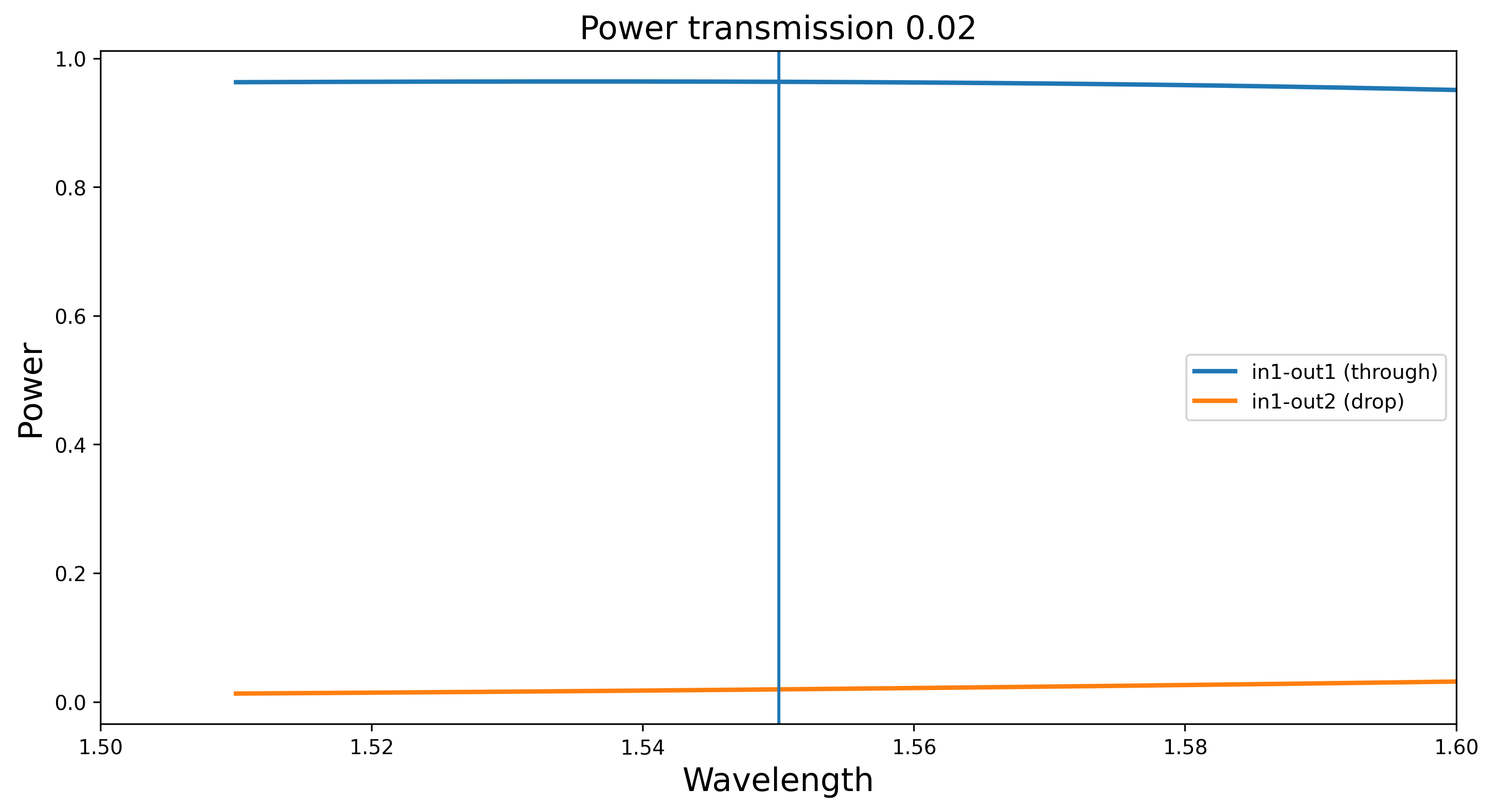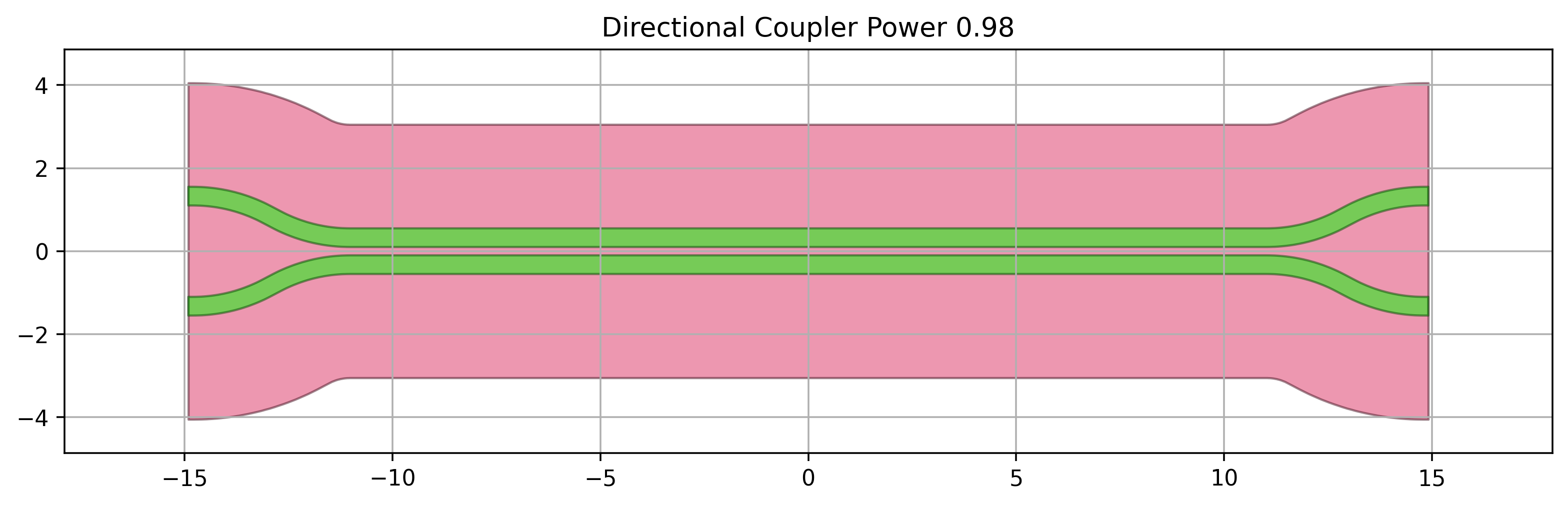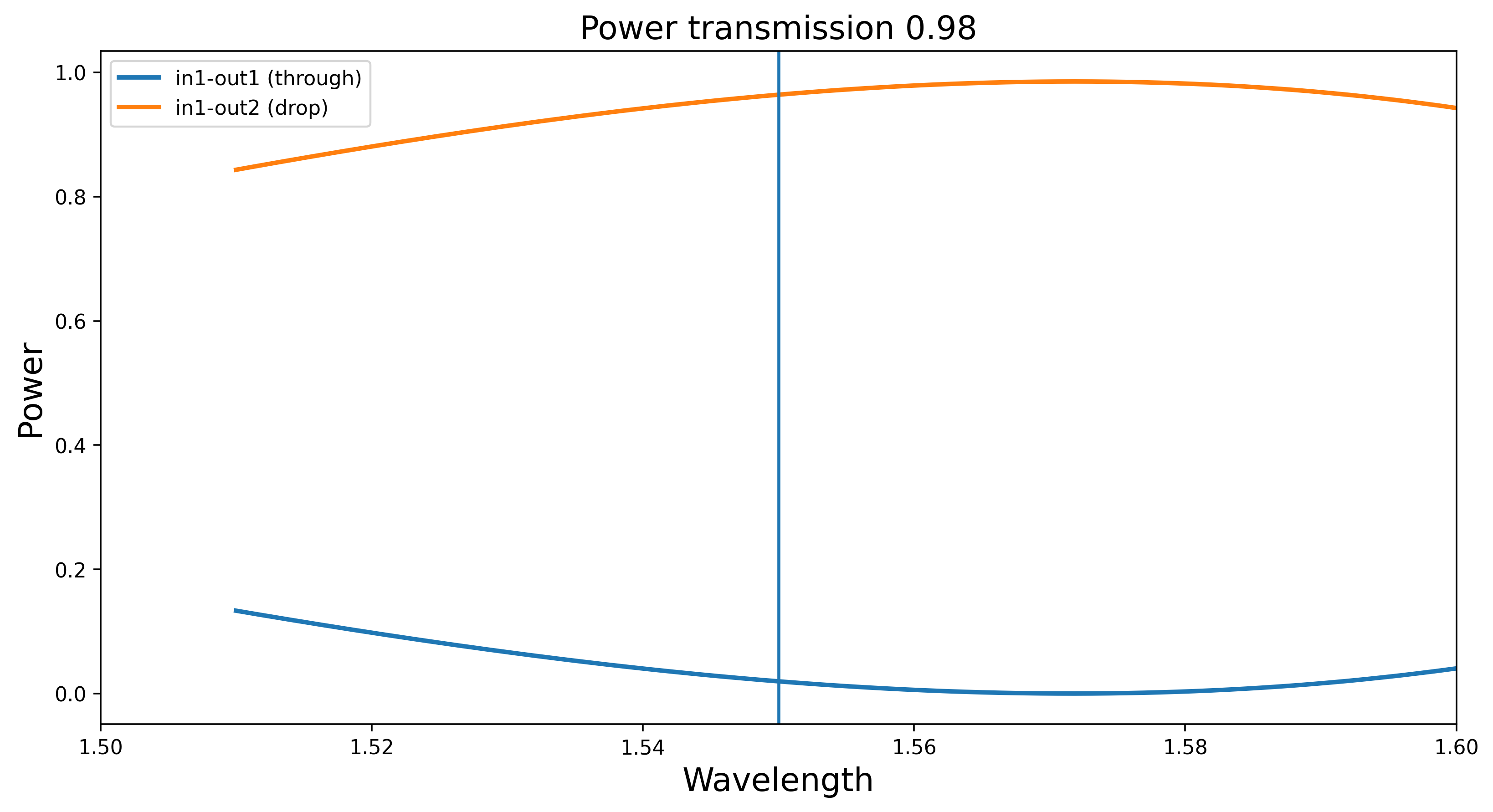In such situations it is advisable to use the adjoint coupling power $$1-p$$ and modify the delay lengths in such a way that the global transfer functions remains the same. Without going into details, we have implemented such a function flip_couplings_and_delays that given a set of power_couplings, delay_lengths and the length_pi, length of a waveguide yielding to a $$\pi$$-phase shift one can compute a new set of coupling factors and delay lengths where the couplers coupling $$p_i$$ will be $$1-p_i$$ for all i in flips.

# Flipping the coefficients and delay lengths
new_couplings, new_delays = flip_couplings_and_delays(couplings=power_couplings_4c,
delay_lengths=delay_lengths_4c,
length_pi=length_pi,
flips=[0, 2])
print """
original_couplings {}
new_couplings {}
original_delays {}
new_delays {}
""".format(power_couplings_4c, new_couplings, delay_lengths_4c, new_delays)

original_couplings [ 0.79338407  0.48366662  0.93601755  0.0613934   0.5       ]
new_couplings [0.20661592613749635, 0.48366662453323794, 0.063982447516684693, 0.061393396497715247, 0.49999999999999989]
original_delays [117.07388265030909, 117.37955858412191, 117.07388265030909, 58.689779292060955]
new_delays [-116.76820671649627, -117.07388265030909, 116.76820671649627, 58.689779292060955]


The longer directional couplers with coupling 0.79 and 0.93 have now been replaced by couplers with coupling 0.2 and 0.07.